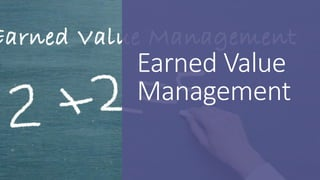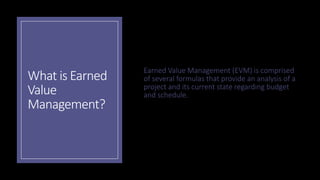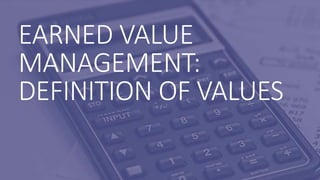Diese Präsentation wurde erfolgreich gemeldet.

# Earned value management (EVM)

Anzeige
Anzeige
Anzeige
Anzeige
Anzeige
Anzeige
Anzeige
Anzeige
Anzeige
Anzeige
Anzeige×

1 von 19 Anzeige

# Earned value management (EVM)

Earned Value Management - Quantifiable project metrics for learning the current state of a project.
Examples and Value Definitions for EVM in relation to project management.

https://agile-mercurial.com
https://twentyfirstcenturyworkforce.com/

Earned Value Management - Quantifiable project metrics for learning the current state of a project.
Examples and Value Definitions for EVM in relation to project management.

https://agile-mercurial.com
https://twentyfirstcenturyworkforce.com/

Anzeige
Anzeige

### Earned value management (EVM)

1. 1. Earned Value Management
2. 2. What is Earned Value Management? Earned Value Management (EVM) is comprised of several formulas that provide an analysis of a project and its current state regarding budget and schedule.
3. 3. EARNED VALUE MANAGEMENT: DEFINITION OF VALUES
4. 4. Budget At Completion (BAC) The Planned Expected Costs to Complete the Project
5. 5. Actual Cost (AC) The actual amount of money spent at a given point
6. 6. Planned Value (PV) The value of the work that should have been completed at any given point for the total project to remain on budget and schedule PV = (Planned Percentage Completed) Multiplied by the Budget At Completion (BAC)
7. 7. Planned Value (PV): Example •Budget At Completion: \$20,000 •Planned Schedule Duration: 100 Days •Current Day in the Project: 15 Days •Actual Work Completed: 12% •Actual Costs (AC): \$3,000 •Planned Percent Completed: 15 Days / 100 Days = 0.15 = 15% 15% X \$20,000 = \$3,000 Planned Value = \$3,000
8. 8. Earned Value (EV) The estimated value of the work completed at any given point EV = (Actual Percentage Completed) X BAC
9. 9. Earned Value (EV): Example •Budget At Completion: \$20,000 •Planned Schedule Duration: 100 Days •Current Day in the Project: 15 Days •Actual Work Completed: 12% •Actual Costs (AC): \$3,000 •Planned Percent Completed: 15 Days / 100 Days = 0.15 = 15% •Planned Value: \$3,000 12% X \$20,000 = \$2,400 Earned Value = \$2,400
10. 10. Cost Variance (CV) The difference between the Earned Value (EV) and the Actual Cost (AC) that shows how much ahead or behind in the budget the project is at any given point A negative CV is the amount the project is over budget A positive CV is the amount the project is under budget
11. 11. Cost Variance (CV): Example •Budget At Completion: \$20,000 •Planned Schedule Duration: 100 Days •Current Day in the Project: 15 Days •Actual Work Completed: 12% •Actual Costs (AC): \$3,000 •Planned Percent Completed: 15 Days / 100 Days = 0.15 = 15% •Planned Value: \$3,000 •Earned Value: \$2,400 \$2,400 - \$3,000 = -\$600 Cost Variance = -\$600 This Project is over budget
12. 12. Cost Performance Index (CPI) An indicator into the speed or rate of spending compared to the value being generated (Is the project budget on track) CPI less than 1 shows the project is spending too fast and is over budget CPI equal to 1 shows the project budget is on track CPI greater than 1 shows the project is under budget Cost Performance Index = Earned Value Divided by Actual Cost
13. 13. Cost Performance Index (CPI): Example •Budget At Completion: \$20,000 •Planned Schedule Duration: 100 Days •Current Day in the Project: 15 Days •Actual Work Completed: 12% •Actual Costs (AC): \$3,000 •Planned Percent Completed: 15 Days / 100 Days = 0.15 = 15% •Planned Value: \$3,000 •Earned Value: \$2,400 •Cost Variance: -\$600 \$2,400/\$3,000 = 0.8 Cost Performance Index = 0.8 This Project is over budget
14. 14. Schedule Variance (SV) The difference between the planned work completed versus the amount of work that was completed A negative SV is an estimate of how much the project is behind schedule A positive SV is an estimate of how much the project is ahead of schedule Schedule Variance = Earned Value – Planned Value
15. 15. Schedule Variance (SV): Example •Budget At Completion: \$20,000 •Planned Schedule Duration: 100 Days •Current Day in the Project: 15 Days •Actual Work Completed: 12% •Actual Costs (AC): \$3,000 •Planned Percent Completed: 15 Days / 100 Days = 0.15 = 15% •Planned Value: \$3,000 •Earned Value: \$2,400 •Cost Variance: -\$600 •Cost Performance Index: 0.8 \$2,400 - \$3,000 = -\$600 Schedule Variance = -\$600 This Project is \$600 worth of work behind schedule
16. 16. Schedule Performance Index (SPI) The indicator into the speed or rate of the work being getting completed compared to the work that was expected to be completed SPI less than 1 shows the project as being behind schedule SPI equal to 1 shows the project as being on track SPI greater than 1 shows that the project is ahead of schedule Schedule Performance Index = Earned Value divided by Planned Value
17. 17. Schedule Performance Index (SPI): Example •Budget At Completion: \$20,000 •Planned Schedule Duration: 100 Days •Current Day in the Project: 15 Days •Actual Work Completed: 12% •Actual Costs (AC): \$3,000 •Planned Percent Completed: 15 Days / 100 Days = 0.15 = 15% •Planned Value: \$3,000 •Earned Value: \$2,400 •Cost Variance: -\$600 •Cost Performance Index: 0.8 •Schedule Variance: -\$600 \$2,400/\$3,000 = 0.8 Schedule Performance Index = 0.8 This Project is behind schedule
18. 18. Other EVM Values Estimate At Completion (EAC) • Based on the current spending rate, EAC is an estimate of how much it will actually cost to complete the whole project • EAC = BAC/CPI Estimate To Completion (ETC) • Based on the current spending rate, ETC is an estimate of how much it will actually cost from a specified point forward to complete the project; it is simply the EAC minus the current actual costs • ETC = EAC – AC Variance At Completion (VAC) • The difference between the planned budget (BAC) and the new forecast budget (EAC) • VAC = BAC – EAC To-Complete Performance Index • An estimate of how hard it would be to meet the project’s objectives • TCPI (BAC – EV)/(BAC – AC)
19. 19. Earned Value Management Joshua Render https://agile-mercurial.com https://twentyfirstcenturyworkfor ce.com/